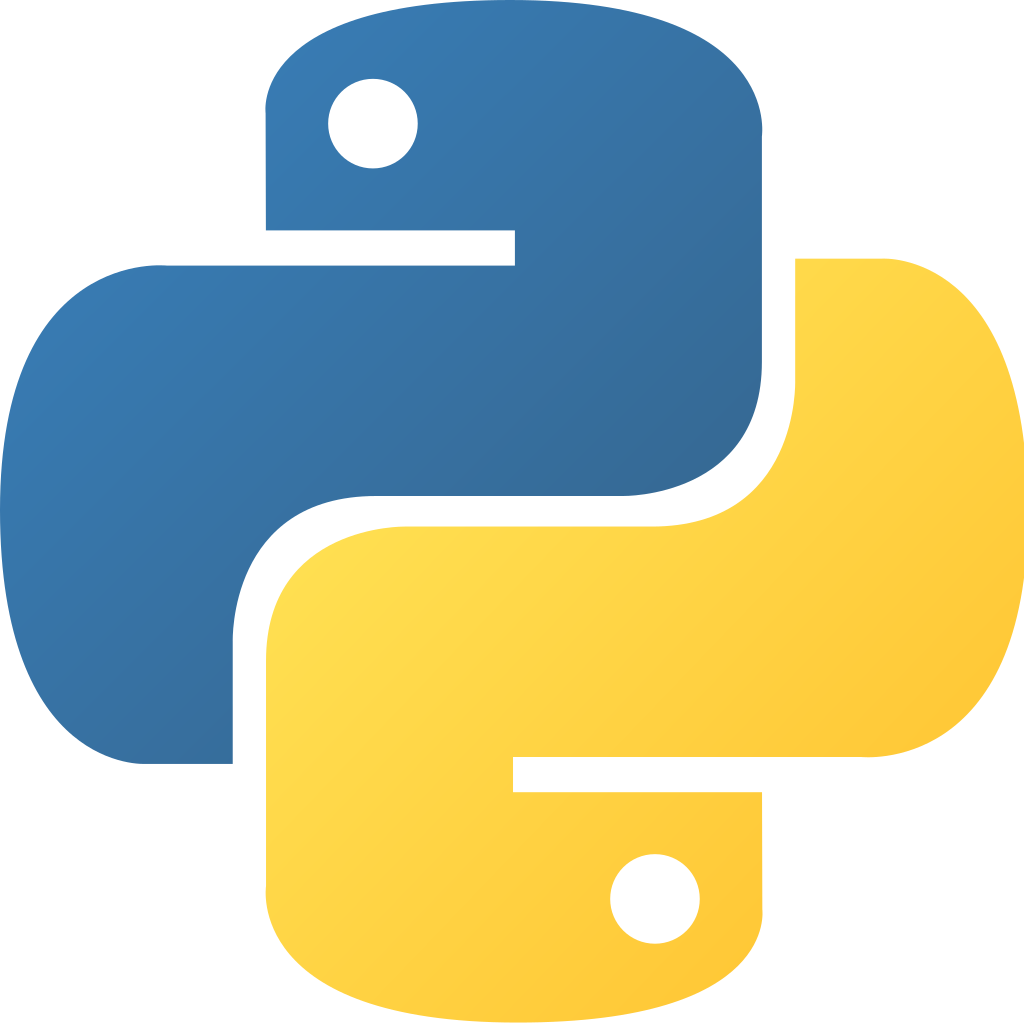Assess your experience with PythonI estimate it would take you 60 steps in 17 minutes to Assess your experience with Python.
When you employ the use of two or more argument specifiers, you include them between opening and close parenthesis, which are called what?
• Statement
• Arch
• Trend
• Tuple
What is the argument specifier for a string?
• %s
• %d
• %f
• %.
What is the argument specifier for a floating point number?
• %d
• %.
• %s
• %f
What is the name of the Python element used to store groups or segments of text?
• Operator
• Variable
• Constant
• String
What is the type of formatting that Python uses to store and create new properly aligned strings?
• Variable Formatting
• Sequential Formatting
• F-Style String Formatting
• C-Style String Formatting
What are the two different types of Python loops called?
• Else and While
• If and While
• If and Then
• For and While
Namespaces that contain multiple groups of Python code and modules are called what?
• Packages
• Operators
• Modules
• Chunks
When variables and functions are combined and encapsulated in a single entity, what are they called?
• Strings
• Objects
• Variables
• Classes
Lists with no duplicate or identical entries are called what?
• Collections
• Sets
• Archives
• Groups
What is the unique way in which Python handles coding errors?
• Condition Highlighting
• Exception Handling
• Sampling
• Code Blocking
💡FUN FACT 💡Python is an interpreted, high-level, general-purpose programming language. Created by Guido van Rossum and first released in 1991, Python's design philosophy emphasizes code readability with its notable use of significant whitespace.
What does it mean that functions are "first-class" in Python?
• Global functions must be defined before classes
• Functions can only be defined in classes
• Methods must accept classes as their first argument
• Functions are objects
What is the exponentiation (power) operator?
• Either ** or ^
• **
• Neither ** or ^
• ^
What is the difference between list comprehensions and generator expressions?
1. There is no difference.

2. List comprehensions produce a list. Generator expressions produce a tuple.

3. List comprehensions produce a list. Generator expressions produce an iterator.

4. List comprehensions produce a list. Generator expressions produce a number.
• 1.
• 2.
• 3.
• 4.
What happens when the conditional expression used with an assert statement is False when evaluated?
• A ProgrammerError is raised.
• An AssertionError is raised.
• The program waits until the expression becomes True.
• The program stops silently.
• Program execution continues as normal.
What is the purpose of triple-quoted strings?
• are used for strings containing regular expressions.
• create strings that can span multiple lines of source code.
• are byte strings.
• are used for strings containing Unicode symbols.
Given: x = not (True or False) What is the value of x?
• False
• 1
• 0
• True
Which of these lines properly determine the number of items in object x?
• count(x)
• x.len()
• x.length()
• len(x)
Given the code:

import math
print("math.pi = {math.pi}, math.e = {math.e}".format(math=math))

What will be displayed?
• math.pi = {math.pi}, math.e = {math.e}
• math.pi = 3.141592653589793, math.e = 2.718281828459045
• math.pi = {3.141592653589793}, math.e = {2.718281828459045}
• A stack trace from the KeyError which will be raised.
How are tuples delimited?
• Parentheses (1, 2, 3)
• Backquotes `1, 2, 3`
• Curly braces {1, 2, 3}
• Square brackets [1, 2, 3]
Which of the following will open a file for editing?
• open("file.txt","wt")
• open("file.txt","et")
• open("file.txt")
• open("file.txt","rt")
💡FUN FACT 💡An important goal of Python's developers is keeping it fun to use. This is reflected in the language's name—a tribute to the British comedy group Monty Python—and in occasionally playful approaches to tutorials and reference materials, such as examples that refer to spam and eggs (from a famous Monty Python sketch) instead of the standard foo and bar.
Given the code:

def change_nth(items, index, value):
items[index] = value
c = [2, 4, 6, 8]
change_nth(c, 1, 5)

What is the value of c ?
• None
• [2, 1, 6, 8]
• [2, 5, 6, 8]
• [2, 4, 6, 8]
Python file objects are iterators. What values does the iterator yield?
• lines of the file.
• bytes from the file.
• Unicode code-points from the file.
• 4 kilobyte chunks from the file.
Which special method can be overridden to customize the setting of attribute values?
• __write__
• __set__
• __setattribute__
• __setattr__
Which file method is used to move the file pointer to the beginning of a file-like object f?
• f.seek()
• f.rewind()
• f.seek(0)
• f.seek(1)
Given the code:

def my_func(*args):
print(args)

How many positional arguments may be passed to my_func?
• Zero
• One
• At least one
• Any amount
What is the standard Python REPL prompt?
• >>>
• >>>>
• \$
• Python>
In an if construct, which one of the following is a valid start to a line that ends with a colon?
• elif
• elsif
• else-if
• elseif
A Python module contains this code:

if __name__ == '__main__':
1. Code that is written with the block after this statement is executed only when the module is directly executed.

2. Code that is written after this statement is ignored by the interpreter, so comments are usually added after it.

3. This code prevents a module from being executed directly.
• 1.
• 2.
• 3.

Or consider 114 other intentions.